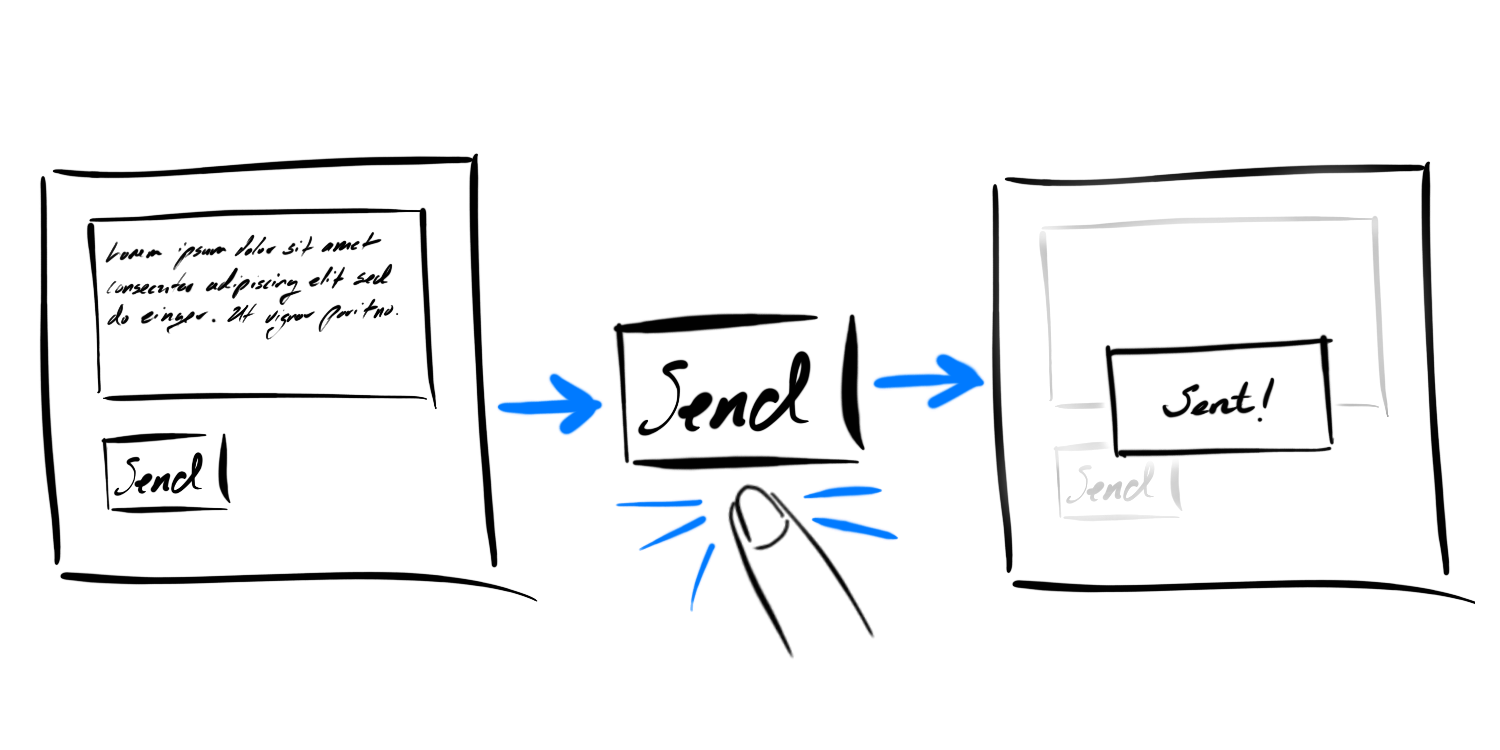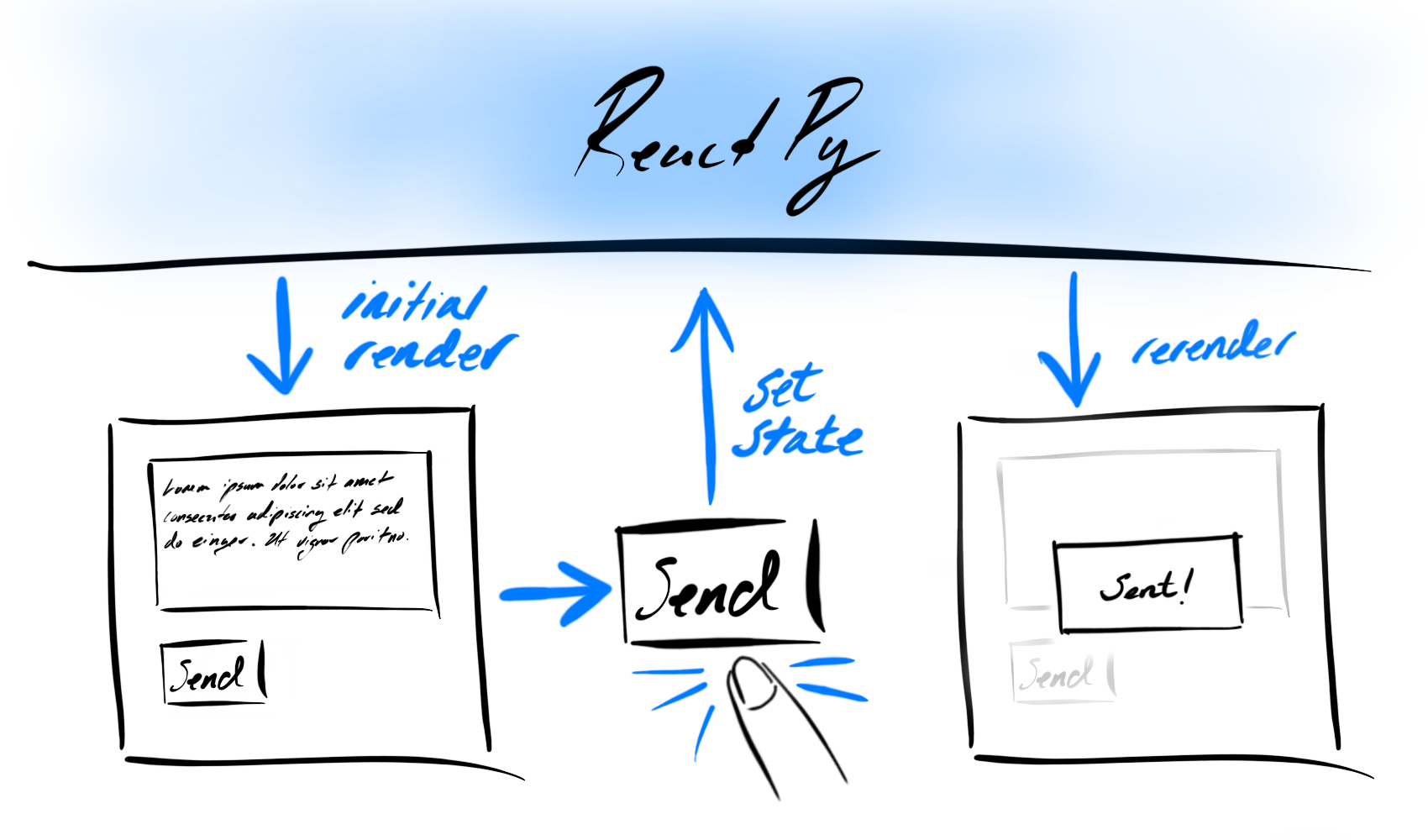# State as a Snapshot#

When you watch the user interfaces you build change as you interact with them, it’s easy to imagining that they do so because there’s some bit of code that modifies the relevant parts of the view directly. As an illustration, you may think that when a user clicks a “Send” button, there’s code which reaches into the view and adds some text saying “Message sent!”:ReactPy works a bit differently though - user interactions cause event handlers to “set state” triggering ReactPy to re-render a new version of the view rather then mutating the existing one.Given this, when ReactPy “renders” something, it’s as if ReactPy has taken a snapshot of the UI where all the event handlers, local variables and the view itself were calculated using what state was present at the time of that render. Then, when user interactions trigger state setters, ReactPy is made away of the newly set state and schedules a re-render. When this subsequent renders occurs it performs all the same calculations as before, but with this new state.

As we’ve already seen, state variables are not like normal variables. Instead, they live outside your components and are managed by ReactPy. When a component is rendered, ReactPy provides the component a snapshot of the state in that exact moment. As a result, the view returned by that component is itself a snapshot of the UI at that time.

## Setting State Triggers Renders#

Setting state does not impact the current render, instead it schedules a re-render. It’s only in this subsequent render that changes to state take effect. As a result, setting state more than once in the context of the same render will not cause those changes to compound. This makes it easier to reason about how your UI will react to user interactions because state does not change until the next render.

Let’s experiment with this behaviors of state to see why we should think about it with respect to these “snapshots” in time. Take a look at the example below and try to guess how it will behave. What will the count be after you click the “Increment” button?

```from reactpy import component, html, run, use_state

@component
def Counter():
number, set_number = use_state(0)

def handle_click(event):
set_number(number + 1)
set_number(number + 1)
set_number(number + 1)

return html.div(
html.h1(number),
html.button({"on_click": handle_click}, "Increment"),
)

run(Counter)
```

Despite the fact that we called `set_count(count + 1)` three times, the count only increments by `1`! This is perhaps a surprising result, but let’s break what’s happening inside the event handler to see why this is happening:

```set_count(count + 1)
set_count(count + 1)
set_count(count + 1)
```

On the initial render of your `Counter` the `number` variable is `0`. Because we know that state variables do not change until the next render we ought to be able to substitute `number` with `0` everywhere it’s referenced within the component until then. That includes the event handler too we should be able to rewrite the three lines above as:

```set_count(0 + 1)
set_count(0 + 1)
set_count(0 + 1)
```

Even though, we called `set_count` three times with what might have seemed like different values, every time we were actually just doing `set_count(1)` on each call. Only after the event handler returns will ReactPy actually perform the next render where count is `1`. When it does, `number` will be `1` and we’ll be able to perform the same substitution as before to see what the next number will be after we click “Increment”:

```set_count(1 + 1)
set_count(1 + 1)
set_count(1 + 1)
```

## State And Delayed Reactions#

Given what we learned above, we ought to be able to reason about what should happen in the example below. What will be printed when the “Increment” button is clicked?

```from reactpy import component, html, run, use_state

@component
def Counter():
number, set_number = use_state(0)

def handle_click(event):
set_number(number + 5)
print(number)

return html.div(
html.h1(number),
html.button({"on_click": handle_click}, "Increment"),
)

run(Counter)
```

If we use the same substitution trick we saw before, we can rewrite these lines:

```set_number(number + 5)
print(number)
```

Using the value of `number` in the initial render which is `0`:

```set_number(0 + 5)
print(0)
```

Thus when we click the button we should expect that the next render will show `5`, but we will `print` the number `0` instead. The next time we click the view will show `10` and the printout will be `5`. In this sense the print statement, because it lives within the prior snapshot, trails what is displayed in the next render.

What if we slightly modify this example, by introducing a delay between when we call `set_number` and when we print? Will this behavior remain the same? To add this delay we’ll use an Async Event Handlers and `sleep()` for some time:

```import asyncio

from reactpy import component, html, run, use_state

@component
def Counter():
number, set_number = use_state(0)

async def handle_click(event):
set_number(number + 5)
await asyncio.sleep(3)
print(number)

return html.div(
html.h1(number),
html.button({"on_click": handle_click}, "Increment"),
)

run(Counter)
```

Even though the render completed before the print statement took place, the behavior remained the same! Despite the fact that the next render took place before the print statement did, the print statement still relies on the state snapshot from the initial render. Thus we can continue to use our substitution trick to analyze what’s happening:

```set_number(0 + 5)
await asyncio.sleep(3)
print(0)
```

This property of state, that it remains static within the context of particular render, while unintuitive at first, is actually an important tool for preventing subtle bugs. Let’s consider the example below where there’s a form that sends a message with a 5 second delay. Imagine a scenario where the user:

1. Presses the “Send” button with the message “Hello” where “Alice” is the recipient.

2. Then, before the five-second delay ends, the user changes the “To” field to “Bob”.

The first question to ask is “What should happen?” In this case, the user’s expectation is that after they press “Send”, changing the recipient, even if the message has not been sent yet, should not impact where the message is ultimately sent. We then need to ask what actually happens. Will it print “You said Hello to Alice” or “You said Hello to Bob”?

```import asyncio

from reactpy import component, event, html, run, use_state

@component
def App():
recipient, set_recipient = use_state("Alice")
message, set_message = use_state("")

@event(prevent_default=True)
async def handle_submit(event):
set_message("")
await asyncio.sleep(5)
print(f"Sent '{message}' to {recipient}")

return html.form(
{"on_submit": handle_submit, "style": {"display": "inline-grid"}},
html.label(
{},
"To: ",
html.select(
{
"value": recipient,
"on_change": lambda event: set_recipient(event["target"]["value"]),
},
html.option({"value": "Alice"}, "Alice"),
html.option({"value": "Bob"}, "Bob"),
),
),
html.input(
{
"type": "text",
"value": message,
"on_change": lambda event: set_message(event["target"]["value"]),
}
),
html.button({"type": "submit"}, "Send"),
)

run(App)
```

As it turns out, the code above matches the user’s expectation. This is because ReactPy keeps the state values fixed within the event handlers defined during a particular render. As a result, you don’t need to worry about whether state has changed while code in an event handler is running.